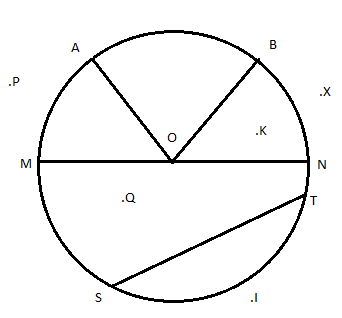EXERCISE4.6.

1) From the figure identify the following.a) The centre of circle                                                                              b) Three radii
Ans. O                                                                                                        Ans: OA, OB, and OC

c) A diameter                                                                                            d) A chord
Ans. AC                                                                                                      Ans: E

e) Two points in the interior                                                                  f) A point in the exterior
Ans: O, P                                                                                                   Ans: Q

g) A sector                                                                                                  h) A segment
Ans: OAB                                                                                                    Ans: ED

a) Is every diameter of a circle also a chord?
Ans: Yes, the diameter is the longest chord of a circle
b) Is every chord of a circle also a diameter?
Ans: No, every chord of the circle is not a diameter

3) Draw any circle and mark
a) Its centre     b) A radius     c) A diameter      d) A sector    e) A segment
f) a point in its interior g) q point in its exterior h) a arca)  The centre is O.                                                                                   b) OS
c) PQ                                                                                                           d) OQ

4) Say true or false:
a) Two diameter of a circle will necessarily intersect.
Ans: True
b) The centre of a circle is always in its interior
Ans: True